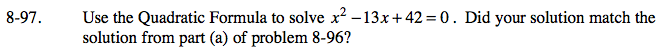### Home > CAAC > Chapter 8 > Lesson 8.3.1 > Problem8-97

8-97.$\textit{x}=\frac{-\textit{b}\pm\sqrt{\textit{b}^2-4\textit{ac}}}{2\textit{a}}$
$\textit{x}=\frac{-(-13)\pm\sqrt{(-13)^2-4(1)(42)}}{2(1)}$
$\frac{13 + \sqrt{1}}{2}\text{ or }\frac{\left(13-\sqrt{1}\right)}{2}$